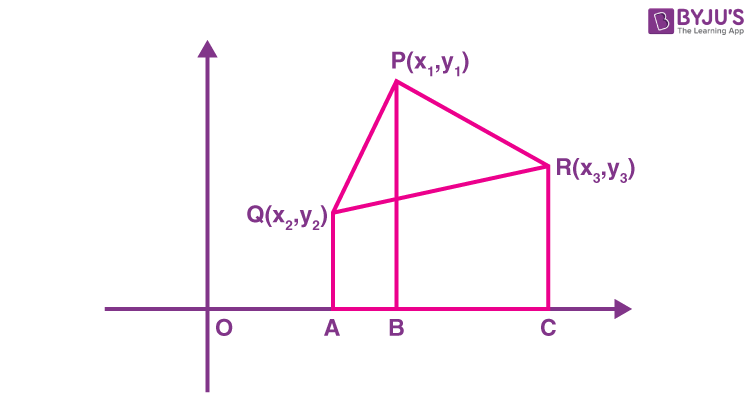# Area of a Triangle In Coordinate Geometry

In Geometry, a triangle is a 3 – sided polygon which has 3 edges and 3 vertices. Area of the triangle is a measure of the space covered by the triangle in the two-dimensional plane. In this article, let us discuss what the area of a triangle is and different methods used to find the area of a triangle in coordinate geometry.

## Methods to Find the Area of a Triangle

Area of a triangle can be found using three different methods. The three different methods are discussed below

### Method 1

When the base and altitude of the triangle are given.

Area of the triangle, A = bh/2 square units

Where b and h are base and altitude of the triangle, respectively.

## Method 2

When the length of three sides of the triangle are given, the area of a triangle can be found using the Heron’s formula.

Therefore, the area of the triangle is calculated using the equation,

$$\begin{array}{l} A = \sqrt{s(s~-~a)~(s~-~b)~(s~-~c)} \end{array}$$

Where a, b, c are the side lengths of the triangle and s is the semi perimeter

The value of s is found using the formula

$$\begin{array}{l} s =\frac {a~+~b~+~c}{2} \end{array}$$

## Method 3

If the vertices of a triangle are given, first we have to find the length of three sides of a triangle. The length can be found using the distance formula.

The procedure to find the area of a triangle when the vertices in the coordinate plane is known.

Let us assume a triangle PQR, whose coordinates P, Q, and R are given as (x1, y1), (x2, y2), (x3, y3), respectively.From the figure, the area of a triangle PQR, lines such as QA, PB and RC are drawn from Q, P and R, respectively perpendicular to x – axis.

Now, three different trapeziums are formed such as PQAB, PBCR and QACR in the coordinate plane.

Now, calculate the area of all the trapeziums.

Therefore, the area of ∆PQR is calculated as, Area of ∆PQR=[Area of trapezium PQAB + Area of trapezium PBCR] -[Area of trapezium QACR] —-(1)

Finding Area of a Trapezium PQAB

We know that the formula to find the area of a trapezium is

Since Area of a trapezium = (1/2) (sum of the parallel sides)×(distance between them)

Area of trapezium PQAB = (1/2)(QA + PB) × AB

QA = y2

PB = y1

AB = OB – OA = x– x2

Area of trapezium PQAB = (1/2)(y+ y2)(x– x2 ) —-(2)

Finding Area of a Trapezium PBCR

Area of trapezium PBCR =(1/2) (PB + CR) × BC

PB = y1

CR = y3

BC = OC – OB =x– x1

Area of trapezium PBCR =(1/2) (y+ y3 )(x– x1) —-(3)

Finding Area of a Trapezium QACR

Area of trapezium QACR = (1/2) (QA + CR) × AC

QA = y2

CR = y3

AC = OC – OA = x– x2

Area of trapezium QACR =(1/2)(y+ y3 ) (x– x2 )—-(4)

Substituting (2), (3) and (4) in (1),

Area of ∆PQR = (1/2)[(y+ y2)(x– x2 ) + (y+ y3 )(x– x1) – (y+ y3 ) (x– x2 )]

A = (1/2) [x1 (y2 – y3 ) + x2 (y– y1 ) + x3(y– y2)]

Special Case:

If one of the vertices of the triangle is the origin, then the area of the triangle can be calculated using the below formula.

Area of a triangle with vertices are (0,0), P(a, b), and Q(c, d) is

A = (1/2)[0(b – d) + a(d – 0) + c(0 – b)]

If area of triangle with vertices P(x1, y1), Q(x2, y2) and R(x3, y3) is zero, then (1/2) [x1 (y2 – y3 ) + x2 (y– y1 ) + x3(y– y2)] = 0 and the points P(x1, y1), Q(x2, y2) and R(x3, y3)are collinear.

### Area of a Triangle in Coordinate Geometry Example

Example: What is the area of the ∆ABC whose vertices are A(1, 2), B(4, 2) and C(3, 5)?

Solution:

Using the formula,

A =  (1/2) [x1 (y2 – y3 ) + x2 (y– y1 ) + x3(y– y2)]

A = (1/2) [1(2 – 5) + 4(5 – 2) + 3(2 – 2)]

A = (1/2) [-3 + 12]=  9/2 square units.

Therefore, the area of a triangle ABC is  9/2 square units.To know more about coordinate geometry and areas of polygons in a coordinate plane, log onto www.byjus.com. To watch interesting videos on the topic, download BYJU’S – The Learning App from Google Play Store.

Quiz on Area of triangle using Coordinates